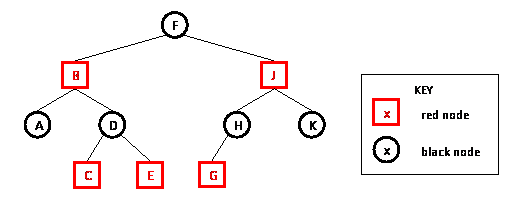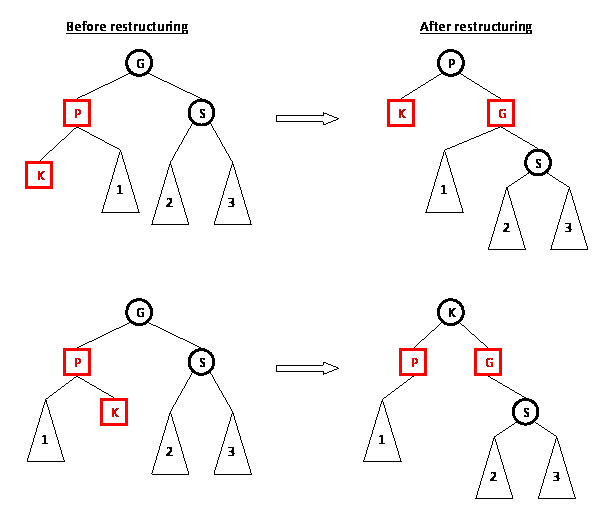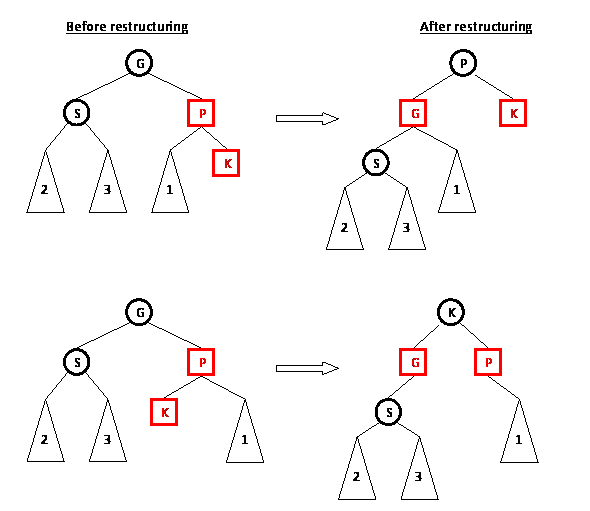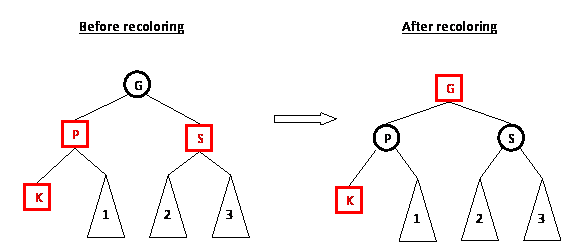# Red-Black Trees

## Introduction

Recall that, for binary search trees, although the average-case times for the lookup, insert, and delete methods are all O(log N), where N is the number of nodes in the tree, the worst-case time is O(N). We can guarantee O(log N) time for all three methods by using a balanced tree -- a tree that always has height O(log N)-- instead of a binary search tree.

A number of different balanced trees have been defined, including AVL trees, 2-4 trees, and B trees. You might learn about the first two in an algorithms class and the third in a database class. Here we will look at yet another kind of balanced tree called a red-black tree.

The important idea behind all of these trees is that the insert and delete operations may restructure the tree to keep it balanced. So lookup, insert, and delete will always be logarithmic in the number of nodes but insert and delete may be more complicated than for binary search trees.

A red-black tree is a binary search tree in which

• each node has a color (red or black) associated with it (in addition to its key and left and right children)
• the following 3 properties hold:
1. (root property) The root of the red-black tree is black
2. (red property) The children of a red node are black.
3. (black property) For each node with at least one null child, the number of black nodes on the path from the root to the null child is the same.

An example of a red-black tree is shown below:## Operations on a Red-Black Tree

As with the binary search tree, we will want to be able to perform the following operations on red-black trees:

• insert a key value (insert)
• determine whether a key value is in the tree (lookup)
• remove key value from the tree (delete)
• print all of the key values in sorted order (print)

Because a red-black tree is a binary search tree and operations that don't change the structure of a tree won't affect whether the tree satisfies the red-black tree properties, the lookup and print operations are identical to lookup and print for binary search trees.

### The insert operation

The goal of the insert operation is to insert key K into tree T, maintaining T's red-black tree properties. A special case is required for an empty tree. If T is empty, replace it with a single black node containing K. This ensures that the root property is satisfied.

If T is a non-empty tree, then we do the following:

1. use the BST insert algorithm to add K to the tree
2. color the node containing K red
3. restore red-black tree properties (if necessary)

Recall that the BST insert algorithm always adds a leaf node. Because we are dealing with a non-empty red-black tree, adding a leaf node will not affect T's satisfaction of the root property. Moreover, adding a red leaf node will not affect T's satisfaction of the black property. However, adding a red leaf node may affect T's satisfaction of the red property, so we will need to check if that is the case and, if so, fix it (step 3). In fixing a red property violation, we will need to make sure that we don't end up with a tree that violates the root or black properties.

For step 3 for inserting into a non-empty tree, what we need to do will depend on the color of K's parent. Let P be K's parent. We need to consider two cases:

Case 1: K's parent P is black

If K's parent P is black, then the addition of K did not result in the red property being violated, so there's nothing more to do.

Case 2: K's parent P is red

If K's parent P is red, then P now has a red child, which violates the red property. Note that P's parent, G, (K's grandparent) must be black (why?). In order to handle this double-red situation, we will need to consider the color of G's other child, that is, P's sibling, S. (Note that S might be null, i.e., G only has one child and that child is P.) We have two cases:

Case 2a: P's sibling S is black or null

If P's sibling S is black or null, then we will do a trinode restructuring of K (the newly added node), P (K's parent), and G (K's grandparent). To do a restructuring, we first put K, P, and G in order; let's call this order A, B, and C. We then make B the parent of A and C, color B black, and color A and C red. We also need to make sure that any subtrees of P and S (if S is not null) end up in the appropriate place once the restructuring is done.

There are four possibilities for the relative ordering of K, P, and G. The way a restructuring is done for each of the possibilities is shown below.

The first two possibilities are:If S is null, then in the pictures above, S (and it's subtrees labelled 2 and 3) would be replaced with nothing (i.e., null).

The second two possibilites are mirror-images of the first two possibilites:Once a restructuring is done, the double-red situation has been handled and there's nothing more to do (you should convince yourself, by looking at the diagrams above, that restructuring will not result in a violation of the black property).

Case 2b: P's sibling S is red

If P's sibling S is red, then we will do a recoloring of P, S, and G: the color of P and S is changed to black and the color of G is changed to red (unless G is the root, in which case we leave G black to preserve the root property).Recoloring does not affect the black property of a tree: the number of black nodes on any path that goes through P and G is unchanged when P and G switch colors (similarly for S and G). But, the recoloring may have introduced a double-red situation between G and G's parent. If that is the case, then we recursively handle the double-red situation starting at G and G's parent (instead of K and K's parent).

An example of adding several values to a red-black tree will be presented in lecture.

#### What is the time complexity for insert?

Inserting a key into a non-empty tree has three steps. In the first step, the BST insert operation is performed. The BST insert operation is O(height of tree) which is O(log N) because a red-black tree is balanced. The second step is to color the new node red. This step is O(1) since it just requires setting the value of one node's color field. In the third step, we restore any violated red-black properties.

Restructuring is O(1) since it involves changing at most five pointers to tree nodes. Once a restructuring is done, the insert algorithm is done, so at most 1 restructuring is done in step 3. So, in the worst-case, the restructuring that is done during insert is O(1).

Changing the colors of nodes during recoloring is O(1). However, we might then need to handle a double-red situation further up the path from the added node to the root. In the worst-case, we end up fixing a double-red situation along the entire path from the added node to the root. So, in the worst-case, the recoloring that is done during insert is O(log N) ( = time for one recoloring * max number of recolorings done = O(1) * O(log N) ).

Thus, the third step (restoration of red-black properties) is O(log N) and the total time for insert is O(log N).

### The delete operation

The delete operation is similar in feel to the insert operation, but more complicated. You will not be responsible for knowing the details of how to delete a key from a red-black tree.

## Summary

Balanced search trees have a height that is always O(log N). One consequence of this is that lookup, insert, and delete on a balanced search tree can be done in O(log N) worst-case time. In constrast, binary search trees have a worst-case height of O(N) and lookup, insert, and delete are O(N) in the worst-case. Red-black trees are just one example of a balanced search tree.

Red-black trees are binary search trees that store one additional piece of information in each node (the node's color) and satisfy three properties. These properties deal with the way nodes can be colored (the root property and the red property) and the number of black nodes along paths from the root node to a null child pointer (the black property). Although it is not intuitively obvious, the algorithms for restoring these properties after a node has been added or removed result in a tree that stays balanced.

While the lookup method is identical for binary search trees and red-black trees, the insert and delete methods are more complicated for red-black trees. The insert method initially performs the same insert algorithm as is done in binary search trees and then must perform steps to restore the red-black tree properties through restructuring and recoloring. The delete method for a red-black tree is more complicated than the insert method and is not included in these notes.